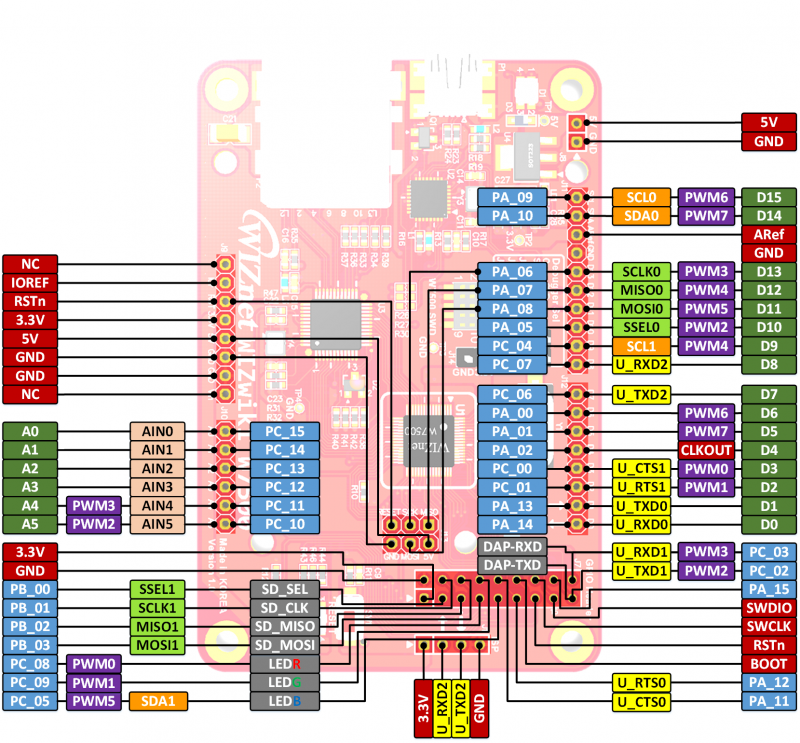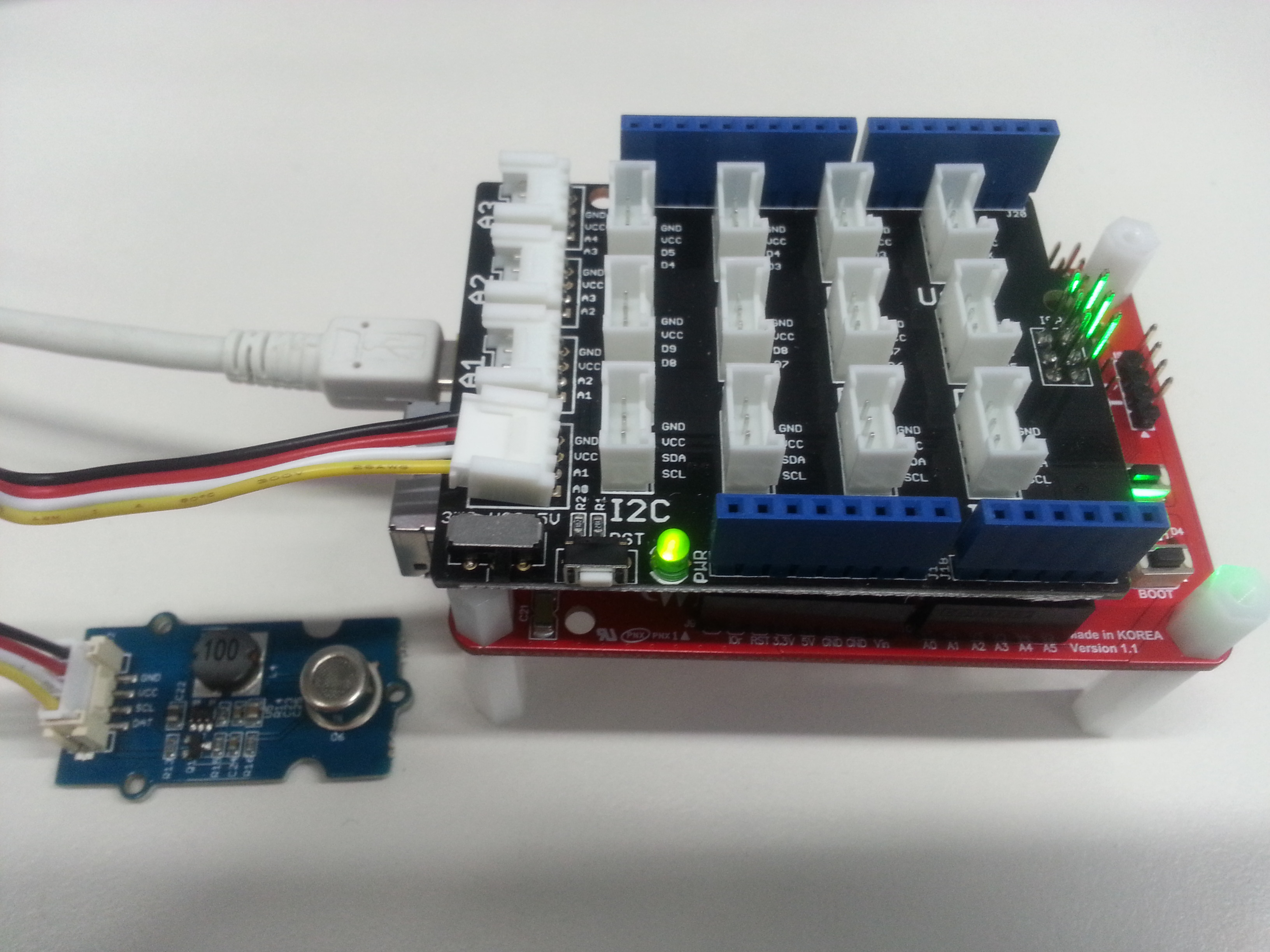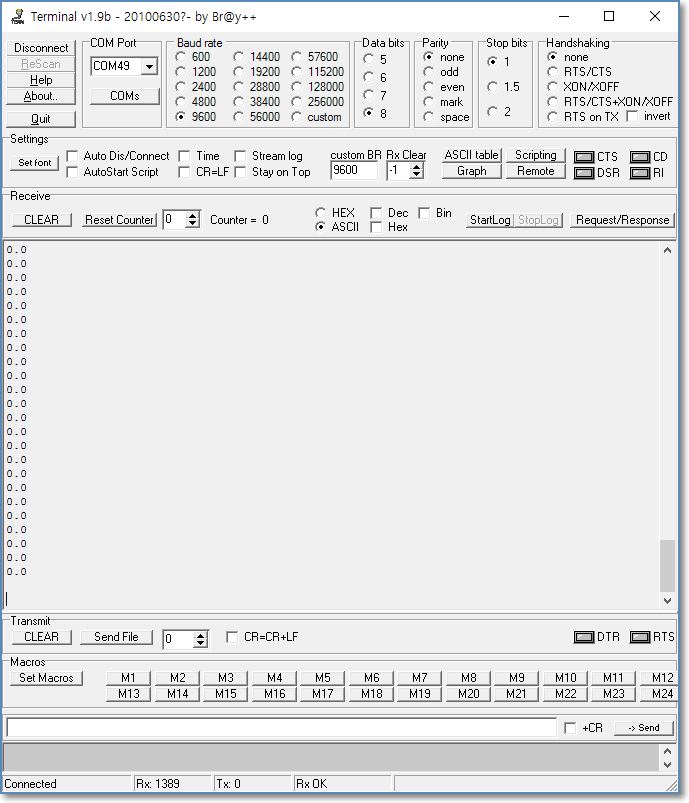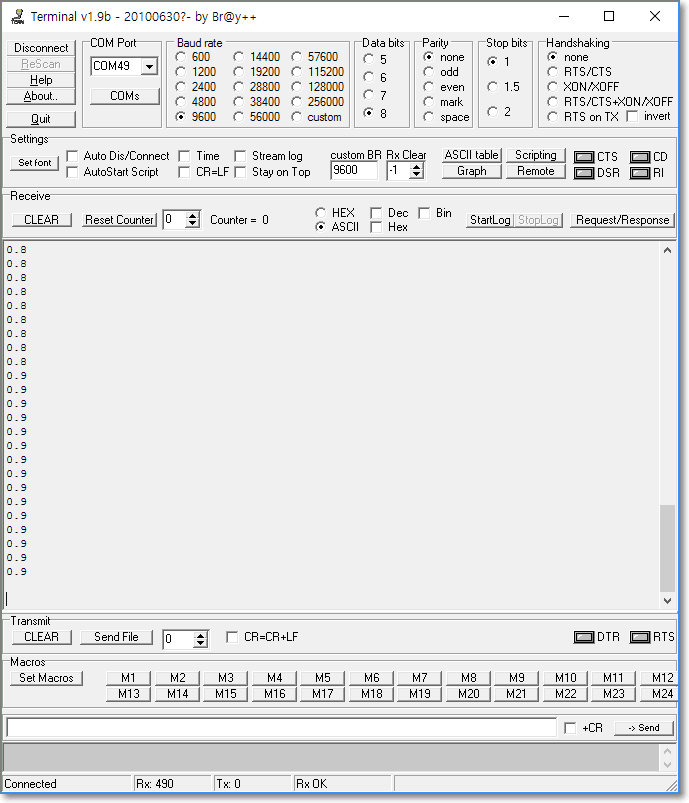Alcohol sensor example with WIZwiki-W7500

Dependencies:   mbed

## Prerequisite

This Program is an example of how to get analog data with WIZwiki-W7500 board A0 Pin.

To implement this function, you need a Platform board only.

## Hardware Configuration

#### WIZwiki-W7500 Pin mapInitialize an analog pin
Initialize an A0 pin of WIZwiki-W7500 platform as an analog pin.

Read analog data from analog pin
Connect an analog device, such as an Alcohol sensor, to A0 pin. And read analog data.

## Software

#### main.cpp

```#include "mbed.h"

DigitalOut heater(A1);
DigitalOut led_r(LEDR);
DigitalOut led_g(LEDG);
DigitalOut led_b(LEDB);

int main(void)
{
float value = 0.0f;

led_g = 0;
led_r = 0;
led_b = 0;

heater = 0;

wait(0.1f);

// Waiting for the sensor to warm-up
while(value > 0.001f) {
wait(1.0f);
value = 1.0f - sensor;
printf("Sensor is warming up : %2.2f\r\n", value);
}
led_g = 1;
led_r = 1;
led_b = 1;

while(1)
{
value = 1- sensor;
printf("%2.1f\r\n", value);
if(value < 0.3)
{
led_g = 0;
led_r = 1;
led_b = 1;
}
else if(value < 0.7)
{
led_g = 1;
led_r = 1;
led_b = 0;
}
else
{
led_g = 1;
led_r = 0;
led_b = 1;
}
wait(1);

}
}
```

## ImagesBefore alcohol testDuring alcohol test